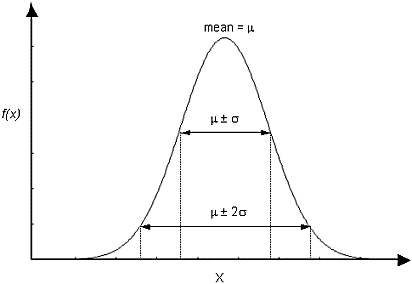# Normal Distribution

The Normal (or Gaussian) Distribution is the most common probability distribution function (PDF) and is generally used for probabilistic studies in geotechnical engineering. Unless there is good reason to use one of the other five PDFs available in RocPlane, it is recommended that you choose the Normal PDF.

For a Normal Distribution, about 68% of observations should fall within one standard deviation of the mean, and about 95% of observations should fall within two standard deviations of the mean.Normal probability density function, showing standard deviation ranges.

## Truncated Normal Distribution

A truncated Normal Distribution can be defined for a variable by setting the desired minimum and/or maximum values for the variable. For practical purposes, minimum and maximum values that are at least three standard deviations away from the mean generate a complete normal distribution. If the minimum / maximum values are less than three standard deviations away from the mean, the distribution is visibly truncated.